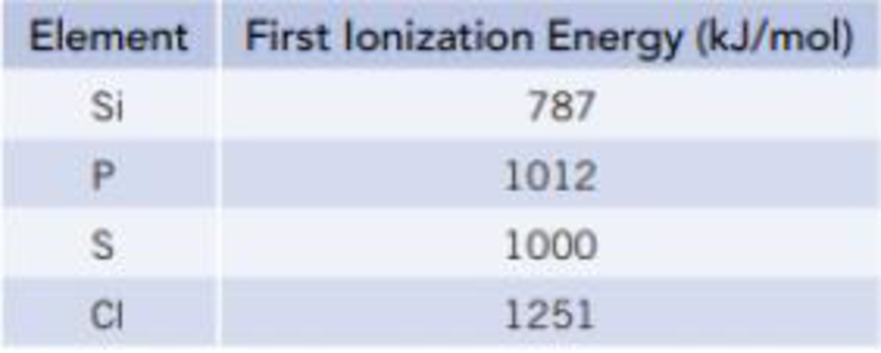Chapter 7, Problem 80SCQ

Chapter
Section
Textbook Problem

The ionization energies for the removal of the first electron in Si, P, S. and CI are as listed in the table below. Briefly rationalize this trend.Interpretation Introduction

Interpretation:

The ionization energy increases along the period; the reason has to be discussed.

Concept Introduction:

Ionization energy (IE): The ionization energy is the required to remove an electron from an atom in the gas phase.

General formula of ionization energy= Atom in the ground state(g)Atom+(g)+eΔU=Ionizationenergy(IE)

As predicted by coulomb’s law, energy must be supplied to overcome the attraction between an electron and the nucleus and to separate the electron from the atom. Thus ionization energies always have positive values. An electron father from the nucleus generally has smaller ionization energy and electron closer to the nucleus has larger ionization energy.

Increase and decrease electro negativity: The less vacancy electrons an atoms has the least it will gain of electrons. Moreover the electron affinity decrease down the groups and from right to left across the periods on the periodic table, the reason is electrons are placed in a higher energy level far from the nucleus thus a decrease from its pull.

Explanation

The decreasing atomic radii is, Si>P>S>Cl

• Analyzing Silicon atom:

Si(g) -Si+(g) + e-[Ne]3s23p2        [Ne]3s23p1

The first ionization energy is based on the how easily an electron can be removed. The removal of electron from partially filled p-orbital is easy and the energy required is less.

Analyzing for Phosphorus (P) atom

P(g) -P+(g) + e-[Lossofoneelectronin3pshells][Ne]3s23p3        [Ne]3s23p2

The removal of electron from half -filled p-orbital is difficult and the energy required is more

Still sussing out bartleby?

Check out a sample textbook solution.

See a sample solution

The Solution to Your Study Problems

Bartleby provides explanations to thousands of textbook problems written by our experts, many with advanced degrees!

Get Started

Find more solutions based on key concepts